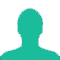# By selling a bicycle for Rs. 2,850, a shopkeeper gains 14%. If the profit is reduced to 8%, then the selling price will be:

A. Rs. 2600

B. Rs. 2700

C. Rs. 2800

D. Rs. 3000

### Solution(By Examveda Team)

Let Cost Price was X.
X + 14% of X = 2850
X + $$\frac{{14{\text{X}}}}{{100}}$$ = 2850
X + 0.14X = 2850
1.14X = 2850
X = 2500.
So, Cost Price = Rs. 2500.
Now, Selling Price When profit remains at 8%,
= 2500 + 8% of 2500
= Rs. 2700.

Short-Cut
CP of bicycle = $$\frac{{100}}{{114}}$$ × 2850 = Rs. 2500
SP for a profit of 8% = $$\frac{{108}}{{100}}$$ × 2500 = Rs. 2700

1.114 percent = 2850 so, 100 percentage =2500
1percentage =25
8 percentage =200
So, 2500 +200=2700rupees

2.c.p=100/(100+14)*2850=2500
if he want to profit 8% on 2500
then s.p=(108/100)*2500=2700

3.,☺☺☺☺☺

4.Salim makes a profit of 15% by selling a cycle at Rs 4140. At what price should he sell it to make a profit of 18% ?
Dimaag h to y answer 10 mins m kar k bataao

5.we have
114% =2850
1%=1/2850
108%=(1/2850)*108=2700

6.plz review the answer this question.answer not fit according to question's instructions.

7.A very good question indeed... :-)

8.how you have do it

Related Questions on Profit and Loss